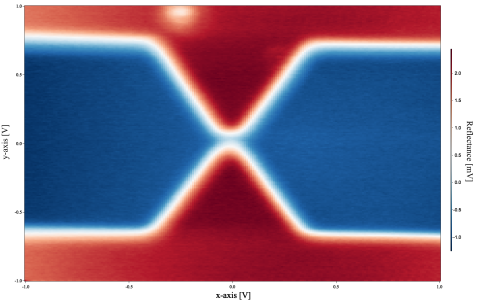# Baseline fit and subtract of an imageHello,

Is there any way to fit and subtract the baseline of an image?
I wrote this function to load the .hdf5 file and plot this image.

Function LoadReflectanceImageFile([variable x_min, variable x_max, variable y_min, variable y_max, variable x_length, variable y_length, string file_name])
x_min = paramIsDefault(x_min) ? -1 : x_min //this means x_min = -1
x_max = paramIsDefault(x_max) ? 1 : x_max //this means x_max = 1
y_min = paramIsDefault(y_min) ? -1 : y_min //this means y_min = -1
y_max = paramIsDefault(y_max) ? 1 : y_max //this means y_max = 1
x_length = paramIsDefault(x_length) ? 201 : x_length //this means x_length = 201
y_length= paramIsDefault(y_length) ? 201 : y_length //this means y_length = 201

Variable groupID
Variable fileID
variable i,j

HDF5OpenFile /R /Z fileID as "" // Displays a dialog

if (V_Flag != 0)
return -1       // User cancelled
endif

if (V_flag == 0)    // User selected a file?
make/o/n=(x_length,1,0,y_length) tempReflectanceImageData
HDF5OpenGroup /Z fileID, "./Traces", groupID
HDF5CloseFile fileID
endif

if(!ParamIsDefault(file_name))
make/o/n=(x_length,y_length) \$file_name
Wave newWave = \$file_name
for(i = 0; i < x_length; i++)
for(j = 0; j< y_length; j++)
newWave[i][j] = tempReflectanceImageData[i][j]
endfor
endfor
showImages(newWave, xLabel="x-axis", yLabel="y-axis", colorLabel="Reflectance")
SetScale/I x x_min,x_max,"", newWave
SetScale/I y y_min,y_max,"", newWave
else
make/o/n=(x_length,y_length) ReflectanceImageData
for(i = 0; i < x_length; i++)
for(j = 0; j< y_length; j++)
ReflectanceImageData[i][j] = tempReflectanceImageData[i][j]
endfor
endfor
showImages(ReflectanceImageData, xLabel="x-axis", yLabel="y-axis", colorLabel="Reflectance")
SetScale/I x x_min,x_max,"", ReflectanceImageData
SetScale/I y y_min,y_max,"", ReflectanceImageData
endif

killwaves tempReflectanceImageData
Label bottom "\\f01\\Z30\\F'Times New Roman' x-axis [V]"
Label left "\\f01\\Z30\\F'Times New Roman' y-axis [V]"
ColorScale/C/N=text0 "\\f01\\Z30\\F'Times New Roman' Reflectance [mV]"

return 0            // Success
End

I have also attached the .pxp & .hdf5 and file.
​​​​​Any help and suggestions are appreciated.

Thanks,

- DohaHello Doha,

I suggest you start by executing the following command:

`DisplayHelpTopic "Background Removal"`

This will take you to a relevant discussion in the ImageProcessing Tutorial that illustrates an approach for solving this problem.

A.G.